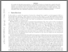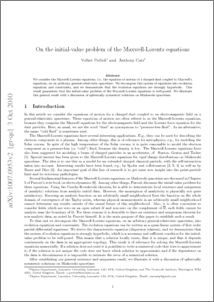# On the initial-value problem of the Maxwell-Lorentz equations.

Perlick, Volker and Carr, Anthony (2010) On the initial-value problem of the Maxwell-Lorentz equations. Journal of Physics A: Mathematical and Theoretical, 43 (44). p. 445502. ISSN 1751-8113Preview
PDF (maxlor.pdf)
maxlor.pdf

## Abstract

We consider the Maxwell-Lorentz equations, i.e., the equation of motion of a charged dust coupled to Maxwell's equations, on an arbitrary general-relativistic spacetime. We decompose this system of equations into evolution equations and constraints, and we demonstrate that the evolution equations are strongly hyperbolic. This result guarantees that the initial-value problem of the Maxwell- Lorentz equations is well-posed. We illustrate this general result with a discussion of spherically symmetric solutions on Minkowski spacetime.

Item Type:
Journal Article
Journal or Publication Title:
Journal of Physics A: Mathematical and Theoretical
Uncontrolled Keywords:
/dk/atira/pure/researchoutput/libraryofcongress/qc
Subjects:
Departments:
ID Code:
34369
Deposited By:
Deposited On:
11 Oct 2010 08:37
Refereed?:
Yes
Published?:
Published